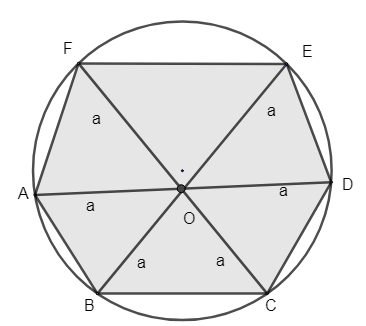QUESTION

# A regular hexagon is inscribed in a circle. If the area of the hexagon is $24\sqrt{3}c{{m}^{2}}$, find the area of the circle. (Use $\pi =3.14$)

Hint: Find the side or radius of the circle by using the area of regular hexagon formula. Area is given so find the side of the hexagon, which is equal to radius of circle. Find the area of the circle.

Consider the hexagon that is drawn. Let us mark the hexagon as ABCDEF. It is said that a regular hexagon is inscribed in a circle. Let the center of the circle be denoted as ‘O’. Let the radius of the circle be denoted as O. Let the radius of the circle be taken as ‘a’. And the side of the hexagon is also ‘a’.We have been given the area of the regular hexagon as $24\sqrt{3}c{{m}^{2}}$.
We need to find the side ‘a’.
We know the formula for hexagon area$=\dfrac{3\sqrt{3}{{a}^{2}}}{2}$
Area of regular hexagon = $24\sqrt{3}$.
$\therefore \dfrac{3\sqrt{3}{{a}^{2}}}{2}=24\sqrt{3}$
Cross multiply and find the value of a.
${{a}^{2}}=\dfrac{24\sqrt{3}\times a}{3\sqrt{3}}=16$
$a=\sqrt{16}=4$cm
Thus we got the side of a regular hexagon, a = 4cm.
We said that the radius of the circle = a = 4cm.
$\therefore$ Area of circle is given by $\pi {{r}^{2}}$, where r is radius which is equal to a.
$\therefore$ Area of circle$=\pi {{a}^{2}}$
\begin{align} & =3.14\times {{4}^{2}} \\ & =3.14\times 4\times 4 \\ & =50.24c{{m}^{2}} \\ \end{align}
Thus we got the area of the circle as $50.24c{{m}^{2}}$.

Note: Formulas to find the area of a hexagon is not something we commonly use. So in case of questions like this remember to learn the formula. Without the formula, you can’t find the sides of the hexagon.Students should remember the formulas for solving these types of problems.